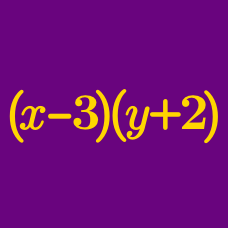Algebra

# Multiplying Polynomials

Which of the following is the correct expansion of $(x+4)(x^2+3) ?$

What is the sum of all the coefficients in the expansion of the expression $(x-2y)(9x-6y-8)?$

If the expansion of $\left(x+\frac{1}{14}y\right)\left(x-\frac{1}{15}y\right)$ gives $x^2+axy+by^2,$ where $a$ and $b$ are constants, what is $\frac{a}{b}?$

The following polynomial in $x$ has a coefficient of $2$ for the quartic (degree four) term: $(3x^4+x^3-19x^2+5x-2k)(x^4+2x^3+3x+k),$ where $k$ is a constant. What is the constant term of the polynomial?

If the expansion of $(x+2y)(Ax+9y)$ gives $3x^2+Bxy+18y^2,$ where $A$ and $B$ are constants, what is $A+B?$

×